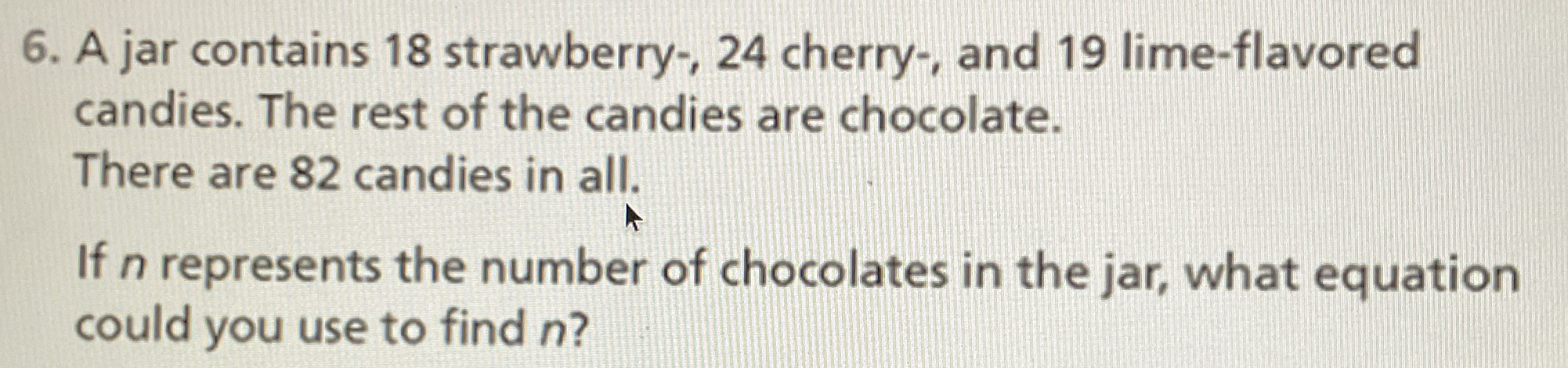### ¿Todavía tienes preguntas de matemáticas?

Pregunte a nuestros tutores expertos
Algebra
PreguntaA jar contains $$18$$ strawberry-, $$24$$ cherry-, and $$19$$ lime-flavored candies. The rest of the candies are chocolate. There are $$82$$ candies in all. If $$n$$ represents the number of chocolates in the jar, what equation could you use to find $$n$$ ?